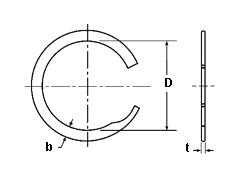### US-131

Display Units: inches |  metric
Request Quick Quote for this part.
 Ring Specs (D) Free Diameter: 1.255 +0.000 / -0.015 in. (t) Thickness: 0.025 +0.002 / -0.002 in. (b) Radial Wall: 0.088 +0.003 / -0.005 in. Groove Specs: (B) Application Diameter: 1.312 in. (G) Groove Diameter: 1.268 +0.004 / -0.004 in. (W) Groove Width: 0.031 +0.002 / -0.000 in. Groove Depth: 0.022 in. Other Specs Approximate Weight per 1000: (m) Material Thickness: 0.024 in. (N) Ring Number of Turns: 1.000 (Pg) Theoretical Thrust Load Capacity - Groove Yield:Notes: Yield Strength of Groove Material (Ys): 45,000 psi. Calculated using a safety factor (K) of 2Equation:Pg = [ B * d * Ys * pi ] / K    = [ (1.312 in.) * (0.022 in.) * (45,000 psi) * 3.14 ] / 2 2,039 lbs. (Pr) Theoretical Thrust Load Capacity - Ring Shear:Notes: Ring Material: Carbon Spring Steel (SAE 1070-1090). Shear Strength of Ring Material (Ss): 126,000 psi. Calculated using a safety factor (K) of 3Equation:Pr = [ B * t * Ss * pi ] / K    = [ (1.312 in.) * (0.025 in.) * (126,000 psi) * 3.14 ] / 3 4,325 lbs. Industry Equivalent Part Number(s):US-131, CL-131, VS-131